# VBA to Convert Text from Lower to Uppercase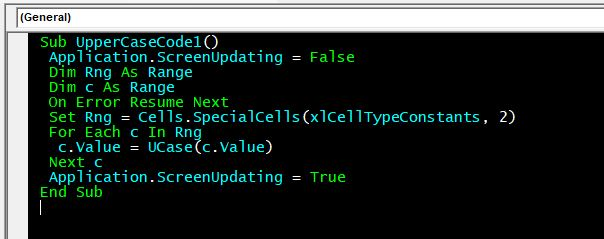We know how to convert lowercase text to uppercase text on worksheet but how do we convert lowercase to uppercase using VBA? While automating the process, you might get the need to convert the text on the sheet to uppercase.

On the worksheet, we use the UPPER function to lowercase to uppecase. In VBA, we have a similar function. The name is the function is UCase. UCase stands for UpperCase.

Syntax of UCase Function:

=UCase(String)

Here string  can be a hard code string or a reference to the string.

So now that we know about the function that can be used to change lowercase letters to uppercase string, let's use it in a practical example.

## Convert All Text On A Sheet to Uppercase

Here we have a simple task to convert all text of a given sheet in a workbook to uppercase. L

To get the code for converting text into upper case letters; we need to follow the steps below to launch VB editor

• Click on the Developer tab
• From Code group, select Visual Basic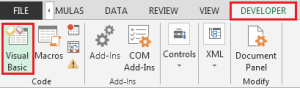Copy the below code to worksheet module (not a normal module).

``` Sub UpperCaseCode1() Application.ScreenUpdating = False Dim Rng As Range Dim c As Range On Error Resume Next Set Rng = Cells.SpecialCells(xlCellTypeConstants, 2) For Each c In Rng c.Value = UCase(c.Value) Next c Application.ScreenUpdating = True End Sub ```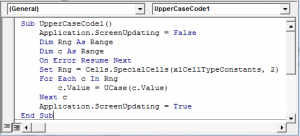How does it work?

The above code is written in the sheet1 module. The above code will convert all the text on the sheet1 to uppercase.

The main lines in the above code are:

```Set Rng = Cells.SpecialCells(xlCellTypeConstants, 2) For Each c In Rng c.Value = UCase(c.Value) Next c ```

Here we use SpecialCells function of Cells class and pass the arguments xlCellTypeConstants. It returns an enumeration of cells and ranges that contain constants.

Next we use the for loop to iterate over each cell in enumeration. Next we change that cell value using UCase function.

Here the statement  `c.Value = UCase(c.Value)` updates the values in each cell with uppercase text.

Example 2: Convert Text of Column A to Uppercase.

In this example, we have the task of converting text column A only. You may want to do this when specific columns contains special text that needs to be in uppercase only.
``` Sub UpperCaseCode2() `````` Application.ScreenUpdating = False Dim cell As Range For Each cell In Range("\$A\$1:" & Range("\$A\$1").SpecialCells(xlLastCell).Address) If Len(cell) > 0 Then cell = UCase(cell) Next cell Application.ScreenUpdating = True End Sub ```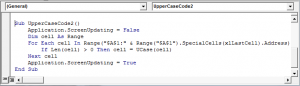The code is almost the same as the previous example. The only change we have is these lines:

```For Each cell In Range("\$A\$1:" & Range("\$A\$1").SpecialCells(xlLastCell).Address) If Len(cell) > 0 Then cell = UCase(cell) Next cell```

Here we create a dynamic range using the line `Range("\$A\$1:" & Range("\$A\$1").SpecialCells(xlLastCell).Address)`. This line creates a range starting from cell A1 to the last cell that contains any value in columna A.

Then we iterate through each cell and update it with uppercase strings of itself.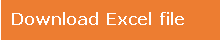Download - VBA To Convert Text From Lower To Uppercase - xlsm

So yeah guys, this is how you can update lowercase text to uppercase text. I hope I was explanatory enough. If  you have any doubts regarding this article or any other VBA related query. Ask me in the comments section below.

Related Articles:

How to Use TRIM function in ExcelThe TRIM function is used to trim strings and clean any trailing or leading spaces from string. This helps us in the cleaning process of data a lot.

How to use the CLEAN function in Excel: Clean function is used to clean up unprintable characters from the string. This function is mostly used with the TRIM function to clean up imported foreign data.

Replace text from end of a string starting from variable positionTo replace text from the end of the string, we use the REPLACE function. The REPLACE function uses the position of text in the string to replace it.

How to Check if a string contains one of many texts in ExcelTo find check if a string contains any of multiple text, we use this formula. We use the SUM function to sum up all the matches and then perform a logic to check if the string contains any of the multiple strings.

Count Cells that contain specific textA simple COUNTIF function will do the magic. To count the number of multiple cells that contain a given string we use the wildcard operator with the COUNTIF function.

Excel REPLACE vs SUBSTITUTE functionThe REPLACE and SUBSTITUTE functions are the most misunderstood functions. To find and replace a given text we use the SUBSTITUTE function. Where REPLACE is used to replace a number of characters in string…

Popular Articles:

50 Excel Shortcuts to Increase Your Productivity | Get faster at your task. These 50 shortcuts will make you work even faster on Excel.

How to use Excel VLOOKUP Function| This is one of the most used and popular functions of excel that is used to lookup value from different ranges and sheets.

How to use the Excel COUNTIF Function| Count values with conditions using this amazing function. You don't need to filter your data to count specific values. Countif function is essential to prepare your dashboard.

How to Use SUMIF Function in Excel | This is another dashboard essential function. This helps you sum up values on specific conditions.

1.Thanks a lot for this explanation?

•Hi Hussain,
You can use events to trigger the macro.
•